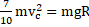# In the figure shown, a small ball of mass ‘’ can move without sliding in a fixed semicircular track of radiusin a vertical plane. It is released from the top. The resultant force on the ball at the lowest point of the track isa)b)c)d) Zero

## Question ID - 100178 :- In the figure shown, a small ball of mass ‘’ can move without sliding in a fixed semicircular track of radiusin a vertical plane. It is released from the top. The resultant force on the ball at the lowest point of the track isa)b)c)d) Zero

3537

 (a) From conservation of energy, the kinetic energy of the ball at the lowest point is (speed of centre of ball)orSince the net tangential force on the sphere at the lowest point is zero, the net force on the sphere at the lowest position isupwards
 A solid sphere of mass, radiusand having moment of inertia about an axis passing through the centre of mass as I, is recast into a disc of thickness, whose moment of inertia about an axis passing through its edge and perpendicular to its plane remains I. Then, radius of the disc will be a)b)c)d)